# Measurement Methods

RFmx SpecAn 19.1 Help

Edition Date: October 2019

Part Number: 374264N-01

»View Product InfoDownload Help (Windows Only)

RFmx SpecAn provides the following methods of measuring the noise figure of a two port DUT.

 Measurement Method Supported Devices Y-Factor PXIe-5668, PXIe-5644/5645/5646*, PXIe-5840*, PXIe 5830/5831* Cold Source PXIe-5644/5645/5646, PXIe-5840, PXIe-5830/31 * Use an external NI Source Measure Unit (SMU) as the noise source power supply for the Noise Figure measurement. Specify the SMU resource name using “NoiseSourcePowerSupply” as the specifier within the RFmxSetup string during RFmx initialization. For example, "RFmxSetup= NoiseSourcePowerSupply:myDCPower" configures RFmx to use channel 0 on myDCPower SMU device for powering the noise source. You should allocate a dedicated SMU channel for RFmx. RFmx supports PXIe-4138 and PXIe-4139 SMUs.

Noise figure measurement consists of two steps, calibration and measurement. Noise figure of the signal analyzer is measured during the calibration step, which is then used in the measurement step.

## Y-Factor Method

The Y-Factor method measures the insertion-gain  and the noise figure of the DUT with the help of a calibrated noise source.

### Noise Source

A calibrated noise source is a broadband noise generator whose level is determined by the effective noise ratio (ENR) specific to the noise source module. The definition of effective noise ratio used by the noise source manufacturers to characterize a noise source is related to the power generated by the noise source when it is turned on.

The following equation describes the ENR specific to the noise source module.where,

THOT is the equivalent noise temperature, in kelvin, of the noise source when it is powered on

T0 is 290 K

The definition of ENR used by the Y-Factor method, as shown in the following equation, is related to the difference between the noise power levels when the noise source is turned on versus when it is turned off.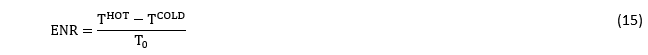TCOLD is the calibration cold temperature specific to each noise source

If the ambient temperature during measurement, TOFF , is different from the calibration cold temperature, TCOLD  of the noise source, the ENR is calculated as shown in the following equation: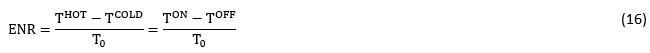### Y-Factor Calibration Step

A calibration step is required to remove the effect of noise generated by the signal analyzer on the reported noise figure of the DUT. In order to perform this calibration, the noise source is connected directly to the RF input port of the signal analyzer and two power measurements are performed, one with the noise source turned on and the other with noise source turned off.

The following figure shows the setup and signal model for NF calibration with a noise source.During the calibration step, two types of losses that need to be considered are the noise source loss and the calibration loss. The signal path for the calibration step is shown in the following figure.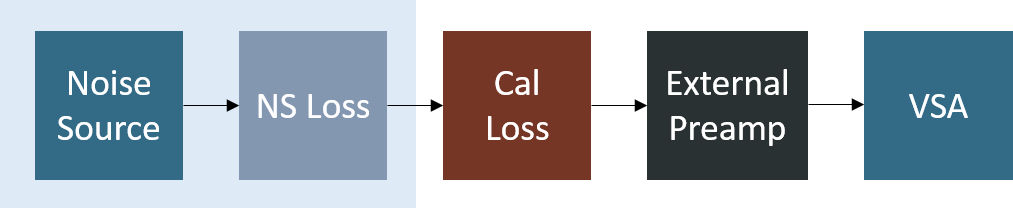The calibration Y-factor is defined as the ratio of the noise power measured when the noise source is turned on to the noise power measured when the noise source is turned off in the absence of the DUT from the signal path. The calibration Y-factor is defined in the following equation using the simplified signal model. Frequency notation in parenthesis is required for frequency converting DUTs, but can be removed for fixed frequency DUTs.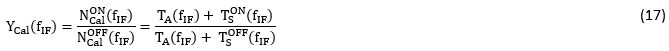Here,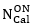and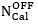are the calibration step noise powers measured when noise source is turned on and off respectively.  TA is the equivalent noise temperature of the signal analyzer, which can be obtained by rearranging equation (17) as shown in the following equation: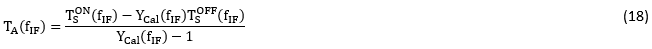### Y-Factor Measurement Step

In the measurement step, the noise source, DUT, and the signal analyzer are cascaded and two power measurements are made; one with the noise source turned on and the other with the noise source turned off.During the measurement step, the three types of losses that needs to be considered are the noise source loss, the DUT input loss, and output loss. The signal path for the measurement step is shown in the following figure.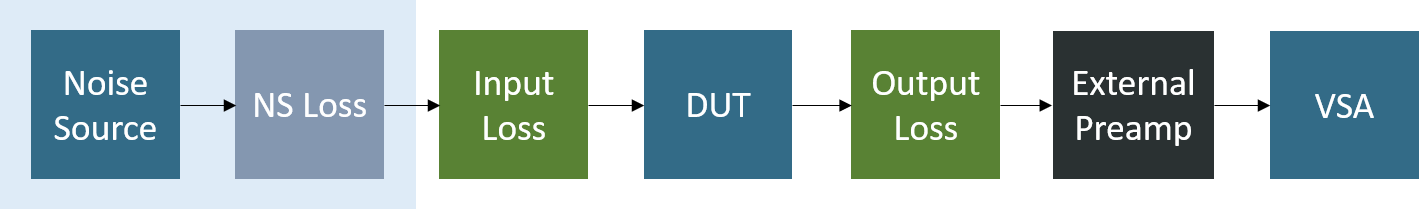Measurement Y-Factor is defined as the ratio of noise power measured when the noise source is turned on to the noise power measured when the noise source is turned off when the DUT is present in the signal path. The measurement Y-factor is defined as shown in the following equation using the simplified signal model. Frequency notation in parenthesis is required for frequency converting DUTs, and can be removed for fixed frequency DUTs.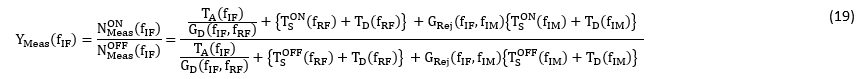For fixed frequency DUTs, the equation simplifies toYou can rearrange this as shown in the following equation: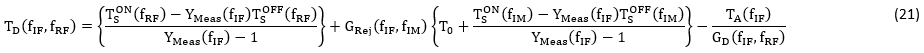For fixed frequency DUTs, the equation simplifies to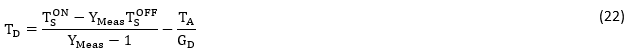The insertion gain, GD, of the DUT is obtained as shown in the following equation: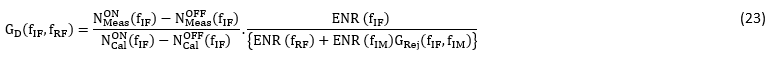For fixed frequency DUTs, the equation simplifies toUsing equations (18), (19), (21), and (22), we can obtain the equivalent noise temperature, TD, of the DUT. Noise figure of the DUT can then be determined in dB as shown in the following equation: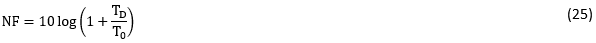## Cold Source Method

Cold source method of measuring noise figure does not rely upon a calibrated noise source. An RF termination is used as the noise source. The signal path for performing a cold source method based noise figure calibration, assuming that the characteristic impedance for the entire signal path is 50 ohms, is shown in the following figure.The signal path for performing a measurement on a DUT, assuming that the characteristic impedance for the entire signal path is 50 ohms, is shown in the following figure.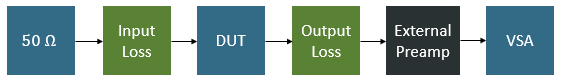Unlike the Y-Factor method, the cold source method does not compute the power gain of the DUT by making power measurements. Instead, this method expects that the S-parameters of the DUT are already available. Using the S-parameters, the NF measurement derives the available-power-gain as shown in the following equations.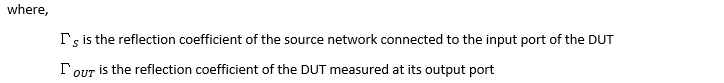where s11, s12, s21, and s22 are the scattering parameters of the DUT. Using the available-power-gain of the DUT and the power measured, noise figure of the DUT is computed.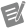Note  Cold source method does not support frequency translating DUTs.

## References

 Egan, William F. Practical RF system design. John Wiley & Sons, 2004.